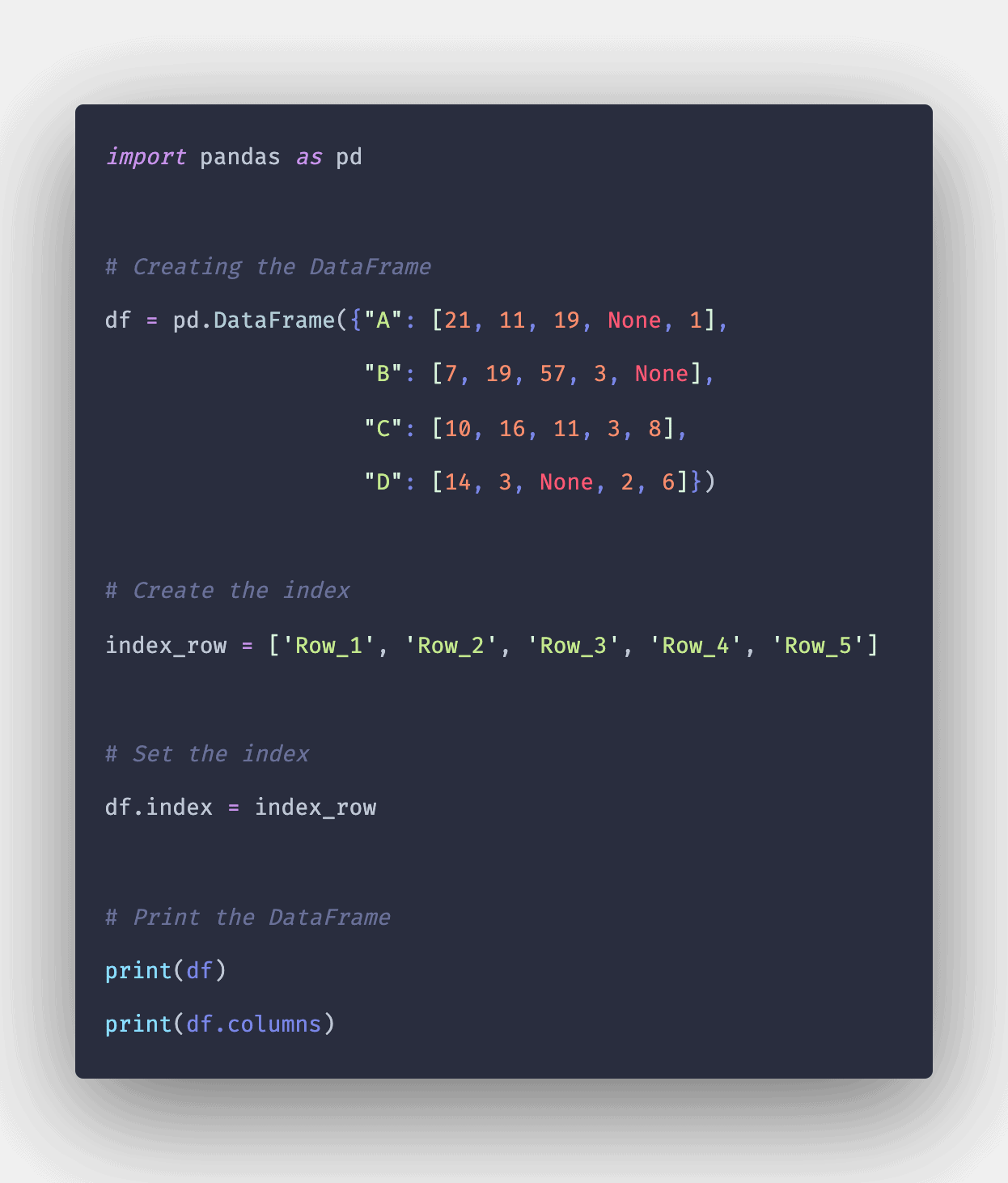# Pandas DataFrame Columns: The Complete Guide

0
76Pandas DataFrame columns are a built-in property used to find the column labels of a given DataFrame. To select a column in Pandas DataFrame, we can access the columns by calling them by their columns name.

DataFrame is in tabular form mostly. Depending on our needs, we can perform many arithmetic operations on the DataFrame on both rows and columns.

## Pandas Columns

To find the column labels of a given DataFrame, use Pandas DataFrame columns property. To deal with columns, we perform basic operations on columns like selecting, deleting, adding, and renaming the columns.

### Syntax

```DataFrame.columns
```

Pandas DataFrame.columns is not a function, which is why it does not have any parameters.

### Return Value

The DataFrame.columns return all the column labels/names of the inputted DataFrame.

### Example program on DataFrame.columns

#### Write a program to show the working of DataFrame.columns.

```import pandas as pd

data = pd.DataFrame({'Name': ['Rohit', 'Raj', 'Shubh', 'Shivam'], 'Marks': [
95, 74, 84, 26], 'Subject': ['Maths', 'Science', 'English', 'Social Science']})
column_names = data.columns
print(column_names)
```

#### Output

`Index(['Name', 'Marks', 'Subject'], dtype='object')`

Here we can see that we created a DataFrame, saved the column names in a variable, and printed the desired column names.

#### Example 2: Create a DataFrame from a dictionary and return the column names of that DataFrame using DataFrame.columns.

See the following code.

```import pandas as pd

data = {'Name': ['Rohit', 'Raj', 'Shubh', 'Shivam'], 'Marks': [95, 74, 84, 26]}
df = pd.DataFrame(data)
column_name = df.columns
print(column_name)
```

#### Output

`Index(['Name', 'Marks'], dtype='object')`

Here we can see that we have first created a dictionary and then used that Dictionary to create a DataFrame after that stored that DataFrame’s column names into a variable and then printed the output.

### Using DataFrame.columns attribute to return the column labels.

We will use the DataFrame.columns attribute to return the column labels of the given DataFrame.

See the following code.

```import pandas as pd

# Creating the DataFrame
df = pd.DataFrame({"A": [21, 11, 19, None, 1],
"B": [7, 19, 57, 3, None],
"C": [10, 16, 11, 3, 8],
"D": [14, 3, None, 2, 6]})

# Create the index
index_row = ['Row_1', 'Row_2', 'Row_3', 'Row_4', 'Row_5']

# Set the index
df.index = index_row

# Print the DataFrame
print(df)
print(df.columns)```

#### Output

```          A     B   C     D
Row_1  21.0   7.0  10  14.0
Row_2  11.0  19.0  16   3.0
Row_3  19.0  57.0  11   NaN
Row_4   NaN   3.0   3   2.0
Row_5   1.0   NaN   8   6.0

Index(['A', 'B', 'C', 'D'], dtype='object')```

As we can see in the output, the DataFrame.columns attribute has successfully returned all of the column labels of the given DataFrame.

## Conclusion

Pandas DataFrame is a two-dimensional, size-mutable, complex tabular data structure with labeled axes (rows and columns). The DataFrame columns attribute returns the column labels of the given Dataframe.

That is it for the Pandas DataFrame columns property.